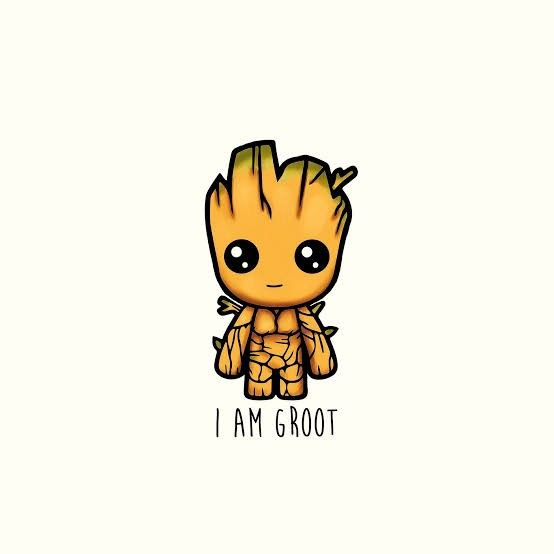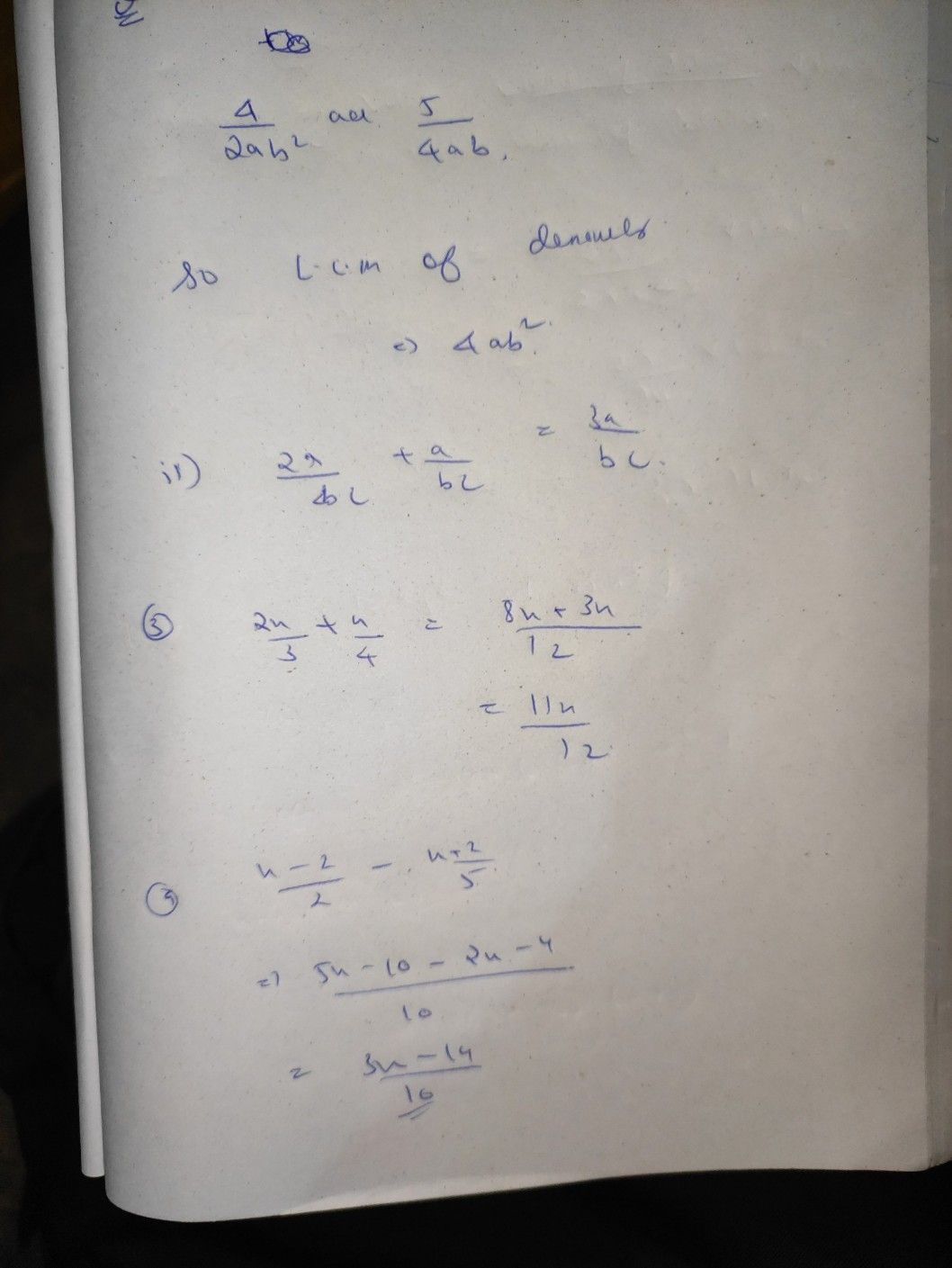Symbol
Problem23 Assessment $Dirθction$ Choose the correct answer. Write your answers on a separate sheet of paper. $1.$ Give the least common denominator $\dfrac {4} {2ab^{2}}$ and 4ab A. ab? C. $4ab2$ B. $2ab2$ D. $6ab^{2}$ 2. $o0kton$ the sum of $\dfrac {2a} {bc}+\dfrac {a} {bc}$ 2bac 2 34ba a c AB. . 3a C. D. be be $3.$ Find simplified $tomα^{\dfrac {2x} {3}+\dfrac {x} {4}}$ $c^{\dfrac {10^{t}x} {12}}$ $0^{\dfrac {11x} {12}}$ A. 19812 x 2 x B. $4.$ Perform the indicated operation. $\dfrac {x-2} {2}-$ $c\dfrac {3x-14} {10}$ A. -3110 x 10 4 B. $x$ $D.-$ as one addend of the sum $\dfrac {8x-2} {3}$ , find the other addend. 5. Given $\dfrac {x+3} {3}$ $\dfrac {6x-4} {3}$ $\dfrac {8x-6} {3}$ A. $c.$
7th-9th grade
Other
Search count: 113
SolutionQanda teacher - AnweshaStudent
Thank you very much